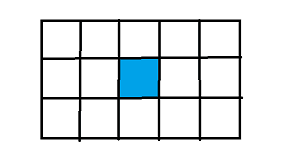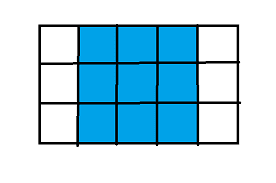Magic Squares
/

## Geometry, Math

Problem
Editorial
Analytics

The problem requires you to find the number of magic squares inside the given rectangle.

Magic squares are the squares inscribed inside the given rectangle whose distance from the top of rectangle to the top of square should be equal to the distance from bottom of the rectangle to the bottom of the square. Also, distance between the left side of the rectangle to left side of the square should be equal to the distance between right side of the rectangle to right side of the square.

eg- For a rectangle with given width 5 and height 3, magic square will be:-Input:

You are given the width and height of the rectangle, respectively.

Output:

Print the total number of distinct magic squares possible.If no such square is possible print -1.

Constraints:

$1 \leq Width,Height \leq 10^{{18}}$

SAMPLE INPUT
5 3
SAMPLE OUTPUT
2
Time Limit: 5.0 sec(s) for each input file.
Memory Limit: 256 MB
Source Limit: 1024 KB

## Contributors

Initializing Code Editor...